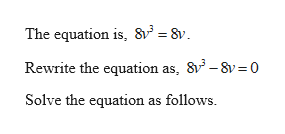# Solve the equation8v3=8v

Question
14 views

Solve the equation

8v3=8v

check_circle

Step 1help_outlineImage TranscriptioncloseThe equation is, 8 = 8v Rewrite the equation as, 83-8v 0 Solve the equation as follows fullscreen

### Want to see the full answer?

See Solution

#### Want to see this answer and more?

Solutions are written by subject experts who are available 24/7. Questions are typically answered within 1 hour.*

See Solution
*Response times may vary by subject and question.
Tagged in

### Algebra